Anzeige

# Energy,heat,work and thermodynamic processes

Student at PEC University of Technology, Chandigarh um PEC University Chandigarh
21. Feb 2017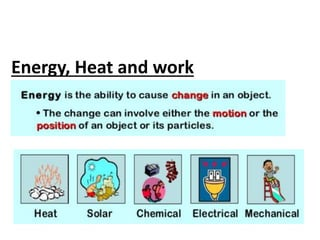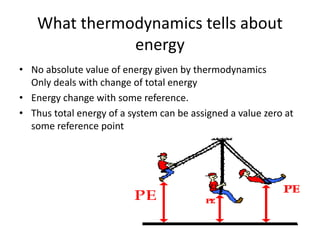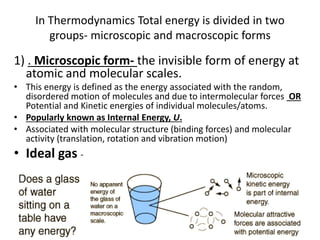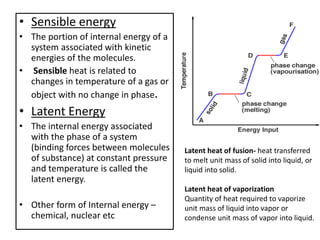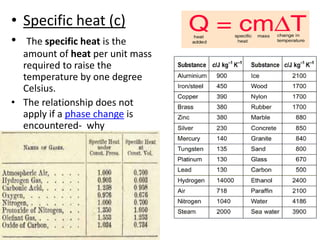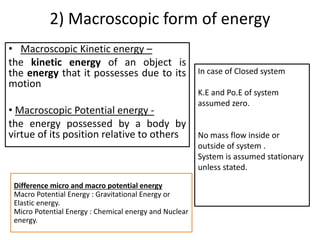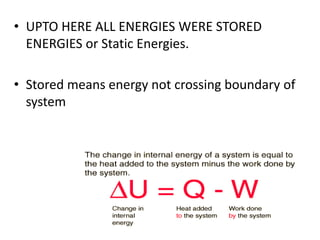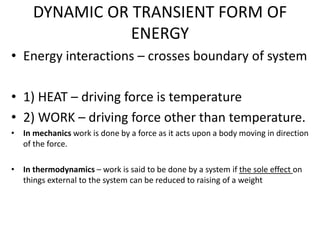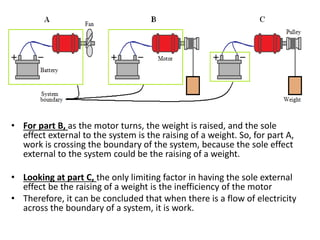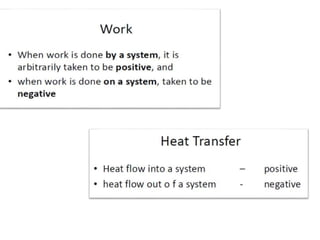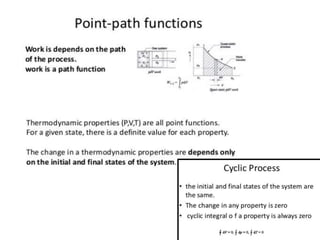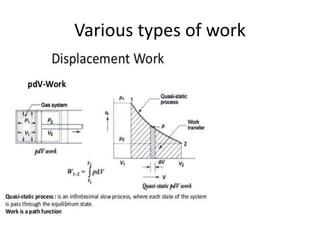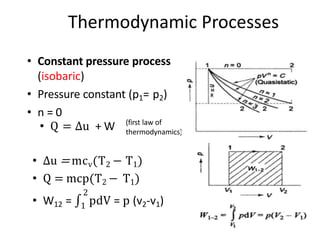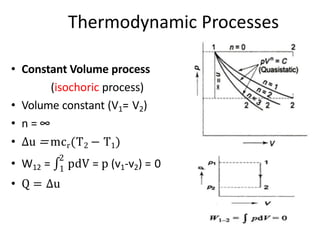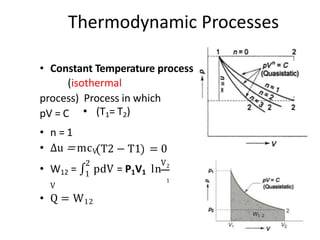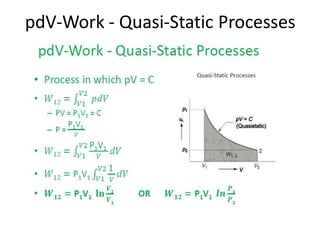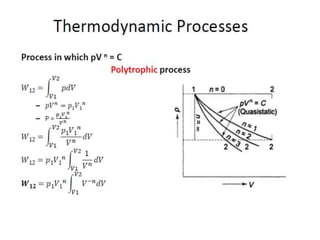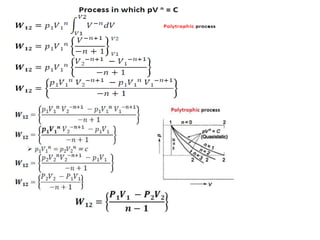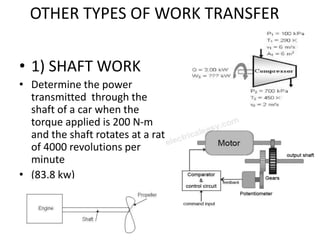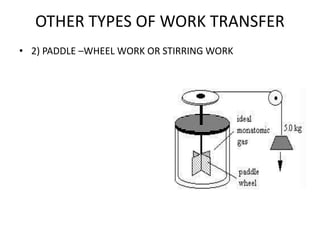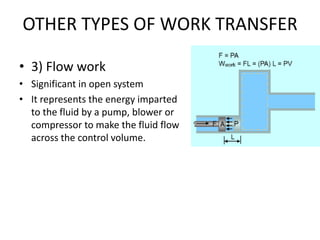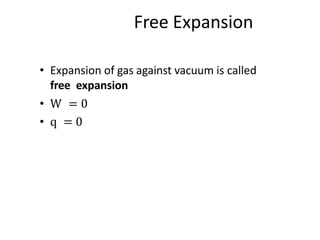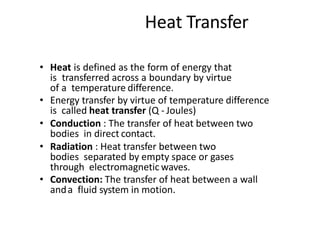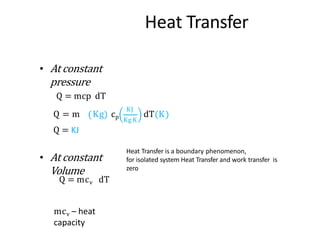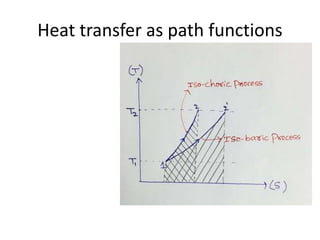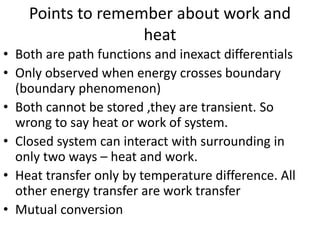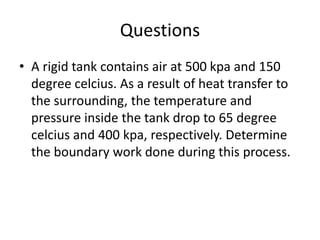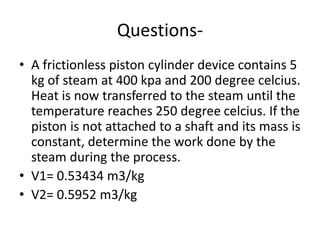1 von 28
Anzeige

### Energy,heat,work and thermodynamic processes

1. Energy, Heat and work What is energy-
2. What thermodynamics tells about energy • No absolute value of energy given by thermodynamics Only deals with change of total energy • Energy change with some reference. • Thus total energy of a system can be assigned a value zero at some reference point
3. In Thermodynamics Total energy is divided in two groups- microscopic and macroscopic forms 1) . Microscopic form- the invisible form of energy at atomic and molecular scales. • This energy is defined as the energy associated with the random, disordered motion of molecules and due to intermolecular forces OR Potential and Kinetic energies of individual molecules/atoms. • Popularly known as Internal Energy, U. • Associated with molecular structure (binding forces) and molecular activity (translation, rotation and vibration motion) • Ideal gas -
4. • Sensible energy • The portion of internal energy of a system associated with kinetic energies of the molecules. • Sensible heat is related to changes in temperature of a gas or object with no change in phase. • Latent Energy • The internal energy associated with the phase of a system (binding forces between molecules of substance) at constant pressure and temperature is called the latent energy. • Other form of Internal energy – chemical, nuclear etc Latent heat of fusion- heat transferred to melt unit mass of solid into liquid, or liquid into solid. Latent heat of vaporization Quantity of heat required to vaporize unit mass of liquid into vapor or condense unit mass of vapor into liquid.
5. • Specific heat (c) • The specific heat is the amount of heat per unit mass required to raise the temperature by one degree Celsius. • The relationship does not apply if a phase change is encountered- why
6. 2) Macroscopic form of energy • Macroscopic Kinetic energy – the kinetic energy of an object is the energy that it possesses due to its motion • Macroscopic Potential energy - the energy possessed by a body by virtue of its position relative to others In case of Closed system K.E and Po.E of system assumed zero. No mass flow inside or outside of system . System is assumed stationary unless stated. Difference micro and macro potential energy Macro Potential Energy : Gravitational Energy or Elastic energy. Micro Potential Energy : Chemical energy and Nuclear energy.
7. • UPTO HERE ALL ENERGIES WERE STORED ENERGIES or Static Energies. • Stored means energy not crossing boundary of system
8. DYNAMIC OR TRANSIENT FORM OF ENERGY • Energy interactions – crosses boundary of system • 1) HEAT – driving force is temperature • 2) WORK – driving force other than temperature. • In mechanics work is done by a force as it acts upon a body moving in direction of the force. • In thermodynamics – work is said to be done by a system if the sole effect on things external to the system can be reduced to raising of a weight
9. • For part B, as the motor turns, the weight is raised, and the sole effect external to the system is the raising of a weight. So, for part A, work is crossing the boundary of the system, because the sole effect external to the system could be the raising of a weight. • Looking at part C, the only limiting factor in having the sole external effect be the raising of a weight is the inefficiency of the motor • Therefore, it can be concluded that when there is a flow of electricity across the boundary of a system, it is work.
10. Various types of work
11. Thermodynamic Processes • Constant pressure process (isobaric) • Pressure constant (p1= p2) • n = 0 • Q = ∆u + W (first law of thermodynamics) 2 • ∆u = mcv(T2 − T1) • Q = mcp(T2 − T1) • W12 = ∫1 pdV = p (v2-v1)
12. Thermodynamic Processes 2 • Constant Volume process (isochoric process) • Volume constant (V1= V2) • n = ∞ • ∆u = mcr(T2 − T1) • W12 = ∫1 pdV = p (v1-v2) = 0 • Q = ∆u
13. Thermodynamic Processes • Constant Temperature process (isothermal process) Process in which pV = C • (T1= T2) • n = 1 • ∆u = mcV 2 T2 − T1 = 0 V2 1 • W12 = ∫1 pdV = P1V1 ln V • Q = W12
14. pdV-Work - Quasi-Static Processes
15. OTHER TYPES OF WORK TRANSFER • 1) SHAFT WORK • Determine the power transmitted through the shaft of a car when the torque applied is 200 N-m and the shaft rotates at a rate of 4000 revolutions per minute • (83.8 kw)
16. OTHER TYPES OF WORK TRANSFER • 2) PADDLE –WHEEL WORK OR STIRRING WORK
17. OTHER TYPES OF WORK TRANSFER • 3) Flow work • Significant in open system • It represents the energy imparted to the fluid by a pump, blower or compressor to make the fluid flow across the control volume.
18. Free Expansion • Expansion of gas against vacuum is called free expansion • W = 0 • q = 0
19. Heat Transfer • Heat is defined as the form of energy that is transferred across a boundary by virtue of a temperature difference. • Energy transfer by virtue of temperature difference is called heat transfer (Q - Joules) • Conduction : The transfer of heat between two bodies in direct contact. • Radiation : Heat transfer between two bodies separated by empty space or gases through electromagnetic waves. • Convection: The transfer of heat between a wall anda fluid system in motion.
20. Heat Transfer • At constant pressure Q = mcp dT KJ Kg K dT(K)Q = m Kg cp Q = KJ • At constant Volume Q = mcv dT mcv – heat capacity Heat Transfer is a boundary phenomenon, for isolated system Heat Transfer and work transfer is zero
21. Heat transfer as path functions
22. Points to remember about work and heat • Both are path functions and inexact differentials • Only observed when energy crosses boundary (boundary phenomenon) • Both cannot be stored ,they are transient. So wrong to say heat or work of system. • Closed system can interact with surrounding in only two ways – heat and work. • Heat transfer only by temperature difference. All other energy transfer are work transfer • Mutual conversion
23. Questions • A rigid tank contains air at 500 kpa and 150 degree celcius. As a result of heat transfer to the surrounding, the temperature and pressure inside the tank drop to 65 degree celcius and 400 kpa, respectively. Determine the boundary work done during this process.
24. Questions- • A frictionless piston cylinder device contains 5 kg of steam at 400 kpa and 200 degree celcius. Heat is now transferred to the steam until the temperature reaches 250 degree celcius. If the piston is not attached to a shaft and its mass is constant, determine the work done by the steam during the process. • V1= 0.53434 m3/kg • V2= 0.5952 m3/kg
Anzeige